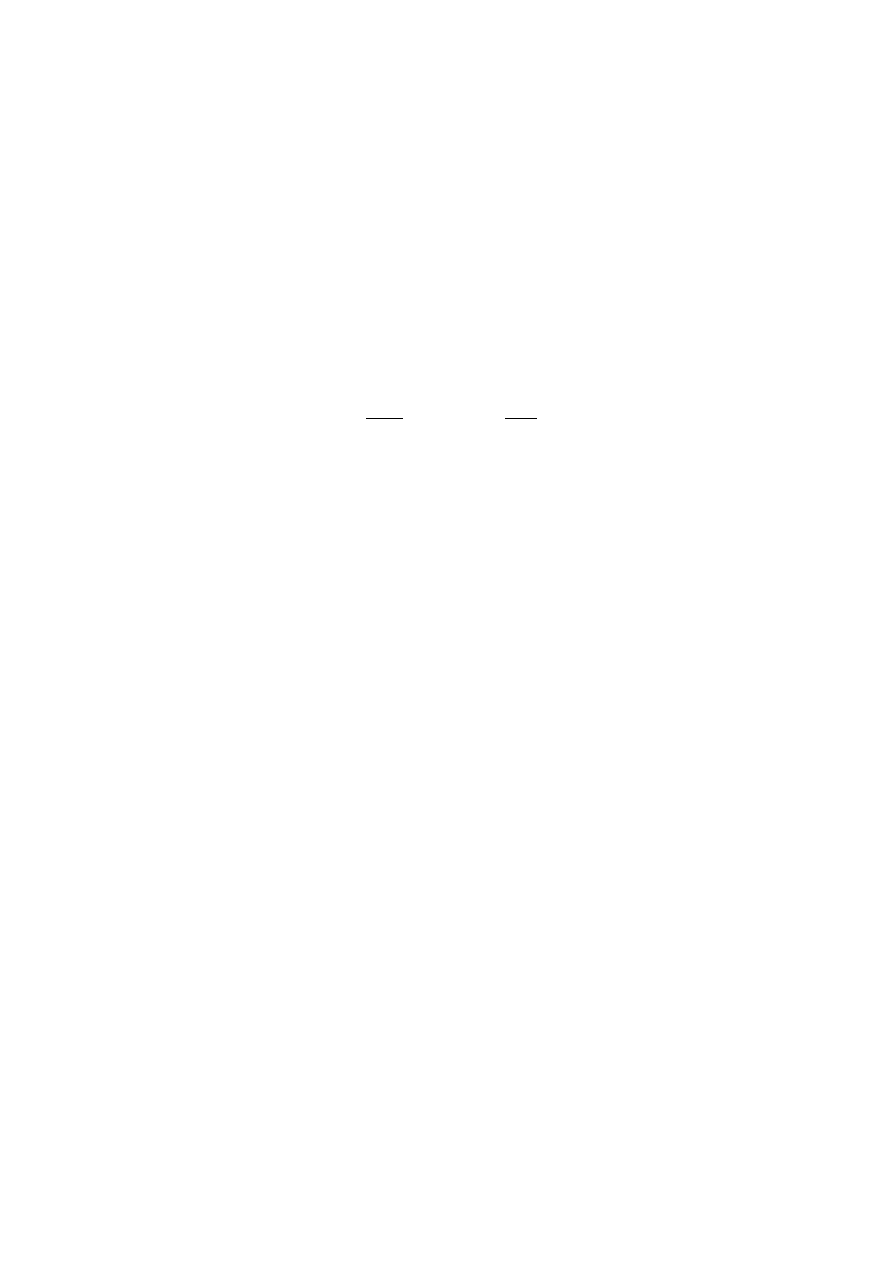ISO/IEC 10918-1 : 1993(E)
Annex A
Mathematical definitions
(This annex forms an integral part of this Recommendation | International Standard)
A.1
Source image
Source images to which the encoding processes specified in this Specification can be applied are defined in this annex.
A.1.1
Dimensions and sampling factors
As shown in Figure A.1, a source image is defined to consist of Nf components. Each component, with unique identifier
C
i
, is defined to consist of a rectangular array of samples of x
i
columns by y
i
lines. The component dimensions are derived
from two parameters, X and Y, where X is the maximum of the x
i
values and Y is the maximum of the y
i
values for all
components in the frame. For each component, sampling factors H
i
and V
i
are defined relating component dimensions x
i
and y
i
to maximum dimensions X and Y, according to the following expressions:
x
X
H
H
y
Y
V
V
i
i
max
i
i
max
=
×
L
M
M
O
P
P
×
L
M
M
O
P
P
and
,
where H
max
and V
max
are the maximum sampling factors for all components in the frame, and
is the ceiling function.
As an example, consider an image having 3 components with maximum dimensions of 512 lines and 512 samples per line,
and with the following sampling factors:
Component
Component 1
Component 2
2
0
4
1
2
2
1
1
0
0
1
1
2
H
V
H
V
H
V
=
=
=
=
=
=
,
,
,
Then X
=
512, Y
=
512, H
max
=
4, V
max
=
2, and x
i
and y
i
for each component are
Component 0
Component
Component
x
y
x
y
x
y
0
0
1
1
2
2
512
256
1
256
512
2
128
256
=
=
=
=
=
=
,
,
,
NOTE ­ The X, Y, H
i
, and V
i
parameters are contained in the frame header of the compressed image data (see B.2.2),
whereas the individual component dimensions x
i
and y
i
are derived by the decoder. Source images with x
i
and y
i
dimensions which do
not satisfy the expressions above cannot be properly reconstructed.
A.1.2
Sample precision
A sample is an integer with precision P bits, with any value in the range 0 through 2
P
­ 1
. All samples of all components
within an image shall have the same precision P. Restrictions on the value of P depend on the mode of operation, as
specified in B.2 to B.7.
A.1.3
Data unit
A data unit is a sample in lossless processes and an
8
×
8
block of contiguous samples in DCT-based processes. The left-
most 8 samples of each of the top-most 8 rows in the component shall always be the top-left-most block. With this top-left-
most block as the reference, the component is partitioned into contiguous data units to the right and to the bottom (as
shown in Figure A.4).
A.1.4
Orientation
Figure A.1 indicates the orientation of an image component by the terms top, bottom, left, and right. The order by which
the data units of an image component are input to the compression encoding procedures is defined to be left-to-right and
top-to-bottom within the component. (This ordering is precisely defined in A.2.) Applications determine which edges of a
source image are defined as top, bottom, left, and right.
24
CCITT Rec. T.81 (1992 E)